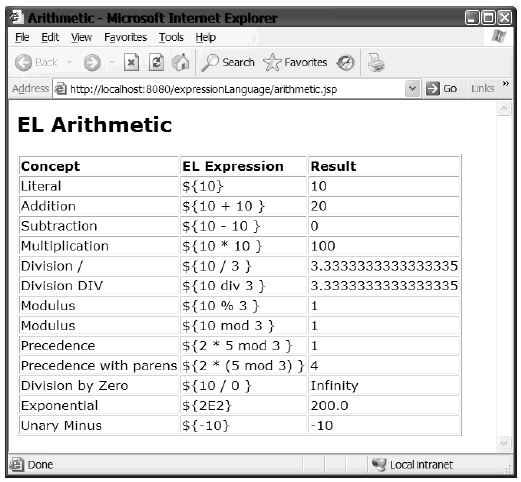# Programming Tutorials

## Arithmetic Evaluation Using the Expression Language in JSP

By: Fazal Printer Friendly Format

This tutorial explains using the EL to evaluate arithmetic operations. There are many cases within web-application development where you need to perform some mathematics on a page. This might be to show a number within the text of a page or to pass a number to a custom tag. In either case, the concepts are exactly the same.

Arithmetic operators are provided to act on both integer and floating-point values. There are six operators that you can use and combine to achieve the vast majority of mathematical calculations with ease:

• Subtraction: -
• Multiplication: *
• Exponents: E
• Division: / or div
• Modulus: % or mod

The last two operators are presented with two alternative syntaxes (both will produce exactly the same result). This is so that the EL is consistent with both the XPath and ECMAScript syntaxes. You can use all the operators in a binary fashion (that is, with two arguments, such as 2 + 3) and the subtraction operator to represent the unary minus (that is, -4 + -2).

As you would expect, each operator has a precedence that determines the order of evaluation of an expression. This precedence is as follows:

• ()
• - (unary)
• * / div mod %
• + - (binary)

You’ll update this list when you look at the comparison operators in the next section. You can, of course, use parentheses to change the order of evaluation, as these take the highest precedence.
With operators of equal precedence, the expression is evaluated from left to right, for example:

2 * 5 mod 3

is equivalent to (2 * 5) mod 3, which evaluates to 1—rather than 2 * (5 mod 3), which evaluates to 4.

Listing below is a JSP page that shows an example of all the operators in action.

arithmetic.jsp
<html>
<title>Arithmetic</title>
<style>
body, td {font-family:verdana;font-size:10pt;}
</style>
<body>
<h2>EL Arithmetic</h2>
<table border="1">
<tr>
<td><b>Concept</b></td>
<td><b>EL Expression</b></td>
<td><b>Result</b></td>
</tr>
<tr>
<td>Literal</td>
<td>\${'\${'}10}</td>
<td>\${10}</td>
</tr>
<tr>
<td>\${'\${'}10 + 10 }</td>
<td>\${10 + 10}</td>
</tr>
<tr>
<td>Subtraction</td>
<td>\${'\${'}10 - 10 }</td>
<td>\${10 - 10}</td>
</tr>
<tr>
<td>Multiplication</td>
<td>\${'\${'}10 * 10 }</td>
<td>\${10 * 10}</td>
</tr>
<tr>
<td>Division / </td>
<td>\${'\${'}10 / 3 }</td>
<td>\${10 / 3}</td>
</tr>
<tr>
<td>Division DIV</td>
<td>\${'\${'}10 div 3 }</td>
<td>\${10 div 3}</td>
</tr>
<tr>
<td>Modulus</td>
<td>\${'\${'}10 % 3 }</td>
<td>\${10 % 3}</td>
</tr>
<tr>
<td>Modulus</td>
<td>\${'\${'}10 mod 3 }</td>
<td>\${10 mod 3}</td>
</tr>
<tr>
<td>Precedence</td>
<td>\${'\${'}2 * 5 mod 3 }</td>
<td>\${2 * 5 mod 3}</td>
</tr>
<tr>
<td>Precedence with parens</td>
<td>\${'\${'}2 * (5 mod 3) }</td>
<td>\${2 * (5 mod 3)}</td>
</tr>
<tr>
<td>Division by Zero</td>
<td>\${'\${'}10 / 0 }</td>
<td>\${10 / 0}</td>
</tr>
<tr>
<td>Exponential</td>
<td>\${'\${'}2E2}</td>
<td>\${2E2}</td>
</tr>
<tr>
<td>Unary Minus</td>
<td>\${'\${'}-10}</td>
<td>\${-10}</td>
</tr>
</table>
</body>
</html>

To run this example, you need to deploy it into a JSP 2.0– or JSP 2.1–compliant web container:

1. Copy the JSP page in Listing 3-3 to the %TOMCAT_HOME%\webapps\expressionLanguage directory.
http://localhost:8080/expressionLanguage/arithmetic.jsp.Figure: shows the output of the JSP page.

The arithemetic operators allow you to perform many basic math operations in a JSP page. All that this JSP page does is print out the result of the expression next to the expression itself. It also demonstrates an interesting technique: that of displaying the \${ characters on a JSP page. This is easily achieved by embedding the literal '\${' in an EL statement. For example, to show the string \${2+3} on a JSP page, you can use the following expression:

\${'\${'}2 + 3 }

You may be thinking that the operators that are provided are not powerful enough. For example, where is the square-root operator? Advanced operators are deliberately not provided to the JSP developer because advanced calculations should not be done in a page. They should either be done in the controller layer of the application, or by using view-helper components such as custom tags or EL functions.

 Most Viewed Articles (in JSP ) Latest Articles (in JSP)

Comment on this tutorial